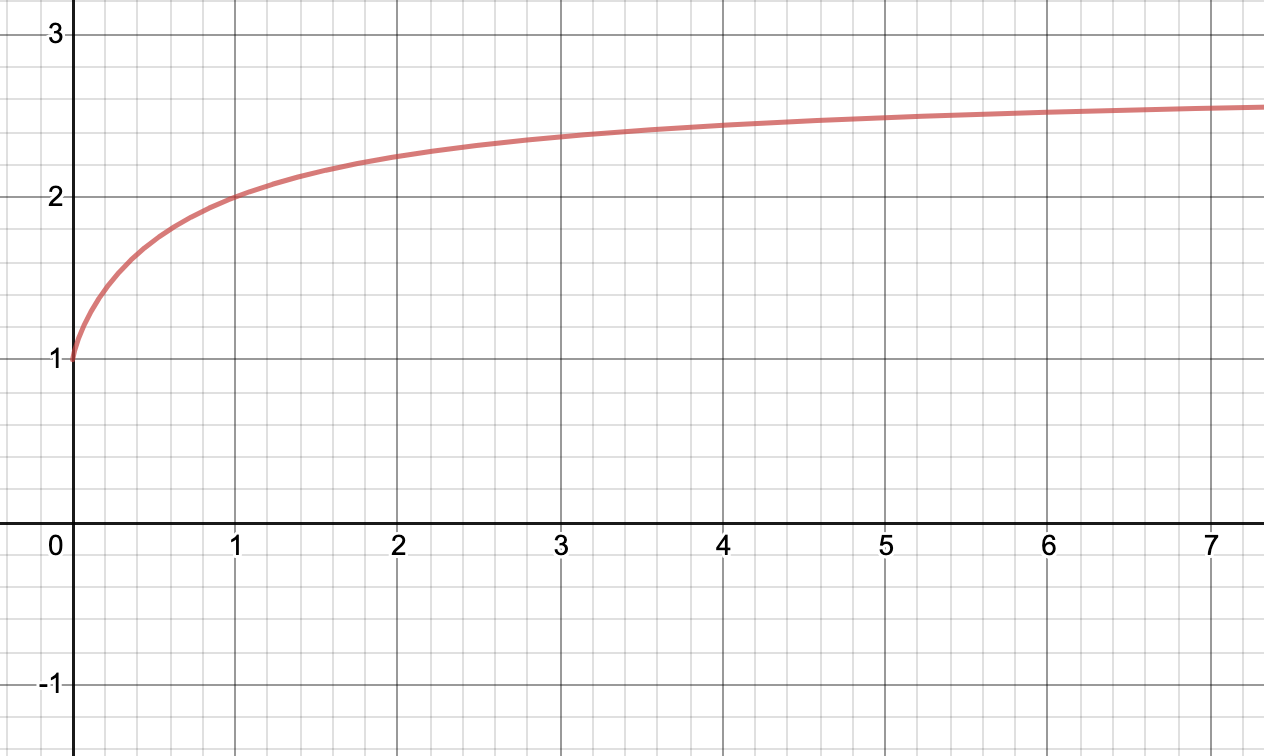# Checking Concavity with Sage

### 09 Mar 2021 - Tags: sage

I haven’t been on MSE lately, because I’ve been fairly burned out from a few weeks of more work than I’d have liked. I’m actually still catching up, with a commutative algebra assignment that should have been done last week. I was (very kindly) given an extension, and I’ll be finishing it soon, though.

I meant to do it today, but I got my second covid vaccination earlier and it really took a lot out of me. I’m feverish and have a pretty bad migraine, so I didn’t want to work on “real things”, but I still wanted to feel productive… MSE it is.

Today someone asked a question, which again I’ll paraphrase here:

Why is $$\left ( 1+\frac{1}{x} \right )^x$$ concave (on $x > 0$)?

It clearly is concave. Here’s a picture of it:Obviously it has an asymptote at $e$, and should always be $\lt e$, so it really should be concave… showing that is a bit of a hassle, though.

Thankfully, we can use sage to automate away most of the difficulties. I’ll more or less be rehashing my answer here. The idea is to put this example of using sage “in the wild” somewhere a bit easier to find than a stray mse post.

Showing that a function is convex (resp. concave) is routine but tedious, especially when that function is twice differentiable. Then we can just check $\frac{d^2f}{dx^2} \geq 0$ (resp. $\leq 0$) on the region of interest. The issue here, of course, is that $\frac{d^2}{dx^2} \left ( 1 + \frac{1}{x} \right )^x$ is… unpleasant. Thankfully, sage doesn’t care in the least! Let’s see if we can bash out the second derivative and show it’s $\leq 0$ (whenever $x > 0$, of course).

We start by defining $f$ and its second derivative

In a perfect world, we could just… ask sage if this is $\leq 0$. Unfortunately, the expression is a bit too complicated, and we don’t get a clean answer1:

This gives a list of lists of domains, and if you intersect all the domains in some fixed list, you get a region where the second derivative is $\lt 0$. Of course, these domains are far too complicated to be useful. We’ll need to try something else.

Let’s look at the second derivative again. We as humans can see how to clean it up a little, so let’s do that first:

$\left ( 1 + \frac{1}{x} \right )^x \left [ \left ( \frac{1}{1+x} - \log \left ( 1 + \frac{1}{x} \right ) \right )^2 - \frac{1}{x^3 + 2x^2 + x} \right ]$

Since $(1 + 1/x)^x$ is always positive when $x$ is, the sign of this expression is controlled by the second factor. We might try to ask sage about the second factor again, but you can check that it’s still too complicated for sage to handle it automatically. We’ll need to simplify the expression if we want to proceed.

One obvious way we might try to simplify things is by turning our expression into a rational function. After all, polynomials are more combinatorial in nature than things like $\log$, and so sage is better equipped to handle them. Your first instinct might be to kill the $\log$s with taylor series, since $x - \frac{x^2}{2} \leq \log(1+x)$. This will work, but we can be a bit more efficient. It’s well known that

$\frac{x}{1+x} \leq \log(1+x) \leq x$

So plugging in $1/x$ and negating, we see

$-\log(1+1/x) \leq - \frac{1}{1+x}$

But that means our expression of interest is upper bounded by

$\left ( \frac{1}{1+x} - \frac{1}{1+x} \right )^2 - \frac{1}{x^3 + 2x^2 + x}$

and we’ve reduced the problem to showing

$- \frac{1}{x^3 + 2x^2 + x} \lt 0 \quad \quad (\text{when } x \gt 0)$

and in the interest of offloading as much thinking as possible to sage, we see

and so $f$ is, in fact, concave.

This was fairly painless, but we got pretty lucky with that estimate for $\log$. I’m curious if there’s a way to completely automate this process, and to remove all need for creativity. If anyone knows a simpler way to do this, where we can just directly ask if the second derivative is negative, I would love to hear about it!

We’re at least a little bit out of luck, since Richardson’s Theorem shows that it’s undecidable whether certain (very nice!) functions are nonnegative. As an easy exercise, can you turn this into a proof that checking convexity is undecidable on some similarly nice class of functions?

Even though logicians came to ruin the fun (as we have a reputation for doing, unfortunately…), I’m curious if any kind of result is possible. Maybe there’s some hacky solution that works fairly well in practice? Approximating every nonpolynomial by the first, say, 50 terms of its taylor series comes to mind, but I’m currently struggling to get sage to expand and simplify expressions in way that makes me happy, so manipulating huge expressions like that is, at least for now, a bit beyond me2.

Again, all ideas are welcome ^_^

1. Another thing I tried was to get sage to do everything for us. But for some reason bool(secondDerivative < 0) returns false, even when we assume(x > 0)… I suspect this is (again) because our expression is too complicated. After all, it seems like there are issues with much simpler expressions than this one. If anyone knows how to make this kind of direct query work, I would love to hear about it!

2. Wow. I know I speak (and write) in run-on sentences, but this one’s on another level. I feel like I need a swear jar but for commas.